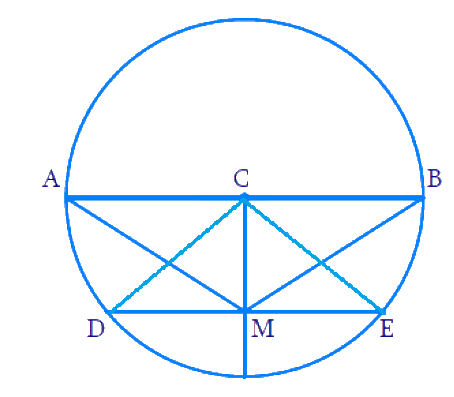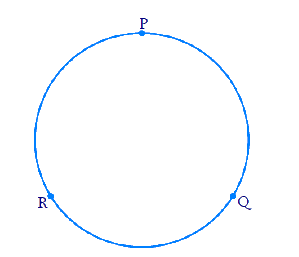NCERT Class 9 Maths Circles

# NCERT Class 9 Maths Circles

This chapter 10 begins with the introduction of circular objects in daily life followed by different terminologies related to circle like radius, diameter, arc, segment, circumference and sectors are stated and explained. Next, the focus shifts on angle subtended by chords at a point, perpendicular from centre to a chord, circle through three points, equal chords and their distance, angle subtended by arc, cyclic quadrilaterals and various theorems based on them. All the concepts are supported by different examples.

Download FREE PDF of Chapter-10 Circles

## Chapter 10 Ex.10.1 Question 1

Fill in the blanks:

(i) The center of the circle lies in            of the circle. (exterior / interior)

(ii) A point, whose distance from the center of circle is greater than its radius lies in                 of the circle. (exterior / interior)

(iii) The longest chord of the circle is a                   of the circle

(iv) An arc is a                  when its ends are the ends of a diameter

(v) Segment of a circle is the region between an arc and                  of the circle

(vi) A circle divides the plane, on which it lies, in                  parts

### Solution

(i) The center of the circle lies in interior of the circle. (exterior / interior)

Reasoning:

The collection of all points in a plane, which are at a fixed distance from a fixed point in the plane, is called a circle. The fixed point is the center of the circle.

(ii) A point, whose distance from the center of circle is greater than its radius lies in exterior of the circle. (exterior / interior)

Reasoning:

The collection of all points in a plane, which are at a fixed distance from a fixed point in the plane is called a circle. The fixed point is the center of the circle. Fixed distance is the radius of the circle. Any point outside the circle will have a greater distance compared to the radius.

(iii) The longest chord of the circle is a diameter of the circle.Reasoning:

Let us check by drawing a random chord \begin{align} {DE}\end{align} and diameter \begin{align} {AB}\end{align} in the circle.

$$AC = CD = CE = BC =$$ radius

$$AB = 2 \times$$ radius

In \begin{align}\Delta\;{DCE},\; {DE}<{DC}+{CE}\end{align} (sum of two sides should be greater than the third side) .

\begin{align} &{DE}\;\lt\;r+r\\ &{DE}\,\lt\;2 r\\&{DE}\;\lt \;{AB}\\&{DE} \;\lt \;\text{diameter}\end{align}

Thus we know that any chord drawn randomly (without passing through the centre) will be shorter than the diameter. Thus Diameter is the longest chord in the circle.

(iv) An arc is a semicircle when its ends are the ends of a diameter.

Reasoning:

We know that diameter is the longest chord in the circle. And diameter divides the circle into $$2$$ equal halves or arcs. When two arcs are equal, each is a semicircle.

(v) Segment of a circle is the region between an arc and chord of the circle.

Reasoning:

The region between a chord and either of its arcs is called a segment of the circular region or simply a segment of the circle.

(vi) A circle divides the plane, on which it lies, in three parts.

Reasoning:

A circle divides the plane on which it lies into three parts.

They are:

(i) inside the circle, which is also called the interior of the circle;

(ii) the circle and

(iii) outside the circle, which is also called the exterior of the circle.

## Chapter 10 Ex.10.1 Question 2

Write True or False: Give reasons for your answers.

### Solution

(i) Line segment joining the center to any point on the circle is the radius of the circle.

Steps:

True

Reasoning:

The collection of all points in a plane, which are at a fixed distance from a fixed point in the plane is called a circle. The fixed point is the center of the circle. Fixed distance is the radius of the circle.

(ii) A circle has only finite number of equal chords.

Steps:

False

Reasoning:

There are infinite points on the circle and hence infinite chords can be drawn between these points.

(iii) If a circle is divided into three equal arcs, each is a major arc.

Steps:

False

Reasoning:If \begin{align} {PQ} \end{align} is a minor arc, then \begin{align} {QRP} \end{align} is a major arc and it should be greater than semicircular arc. If there are three arcs none of it can be a major arc.

(iv) A chord of a circle, which is twice as long as its radius, is a diameter of the circle.

Steps:

True

Reasoning:

Draw a chord that passes through the centre of the circle. We can see that this chord is twice the length of the radius of the circle. This is called the diameter of the circle.

(v) Sector is the region between the chord and its corresponding arc.

Steps:

False

Reasoning:

The region between an arc and the two radii, joining the centre to the end points of the arc is called a sector.

(vi) A circle is a plane figure.

Steps:

True

Reasoning:

A circle is a $$2$$ dimensional figure. So Circle is a plane figure.

Circles | NCERT Solutions
Instant doubt clearing with Cuemath Advanced Math Program